The Australian Journal of Mathematical Analysis and Applications

 Home News Editors Volumes RGMIA Subscriptions Authors Contact

ISSN 1449-5910

You searched for yang
Total of 160 results found in site

11: Paper Source PDF document

Paper's Title:

Existence of Solutions for Third Order Nonlinear Boundary Value Problems

Author(s):

Yue Hu and Zuodong Yang

School of Mathematics and Computer Science, Nanjing Normal University, Jiangsu Nanjing 210097,
China.
huu3y2@163.com

College of Zhongbei, Nanjing Normal University, Jiangsu Nanjing 210046,
China.
zdyang_jin@263.net
yangzuodong@njnu.edu.cn

Abstract:

In this paper, the existence of solution for a class of third order quasilinear ordinary differential equations with nonlinear boundary value problems

p(u"))'=f(t,u,u',u"), u(0)=A, u'(0)=B, R(u'(1),u"(1))=0

is established. The results are obtained by using upper and lower solution methods.

10: Paper Source PDF document

Paper's Title:

On an Extension of Hilbert’s Integral Inequality with Some Parameters

Author(s):

Bicheng Yang

Department of Mathematics, Guangdong Education College, Guangzhou, Guangdong 510303, People’s Republic of China.
URL
:

Abstract:

In this paper, by introducing some parameters and estimating the weight function, we give an extension of Hilbert’s integral inequality with a best constant factor. As applications, we consider the equivalent form and some particular results.

9: Paper Source PDF document

Paper's Title:

A Strengthened Hardy-Hilbert's Type Inequality

Author(s):

Weihong Wang and Bicheng Yang

Department of Mathematics, Guangdong Education Institute,
Guangzhou, Guangdong 520303,
People's Republic Of China
wwh@gdei.edu.cn
bcyang@pub.guangzhou.gd.cn
URL: http://www1.gdei.edu.cn/yangbicheng/index.html

Abstract:

By using the improved Euler-Maclaurin's summation formula and estimating the weight coefficient, we give a new strengthened version of the more accurate Hardy-Hilbert's type inequality. As applications, a strengthened version of the equivalent form is considered.

8: Paper Source PDF document

Paper's Title:

On Positive Entire Solutions of Second Order Quasilinear Elliptic Equations

Author(s):

Zuodong Yang and Honghui Yin

Institute of Mathematics, School of Mathematics and Computer Science,
Nanjing Normal University, Jiangsu Nanjing 210097,
China;
zdyang_jin@263.net

Department of Mathematics, Huaiyin Teachers College,
Jiangsu Huaian 223001,
China;
School of Mathematics and Computer Sciences,
Nanjing Normal University, Jiangsu Nanjing 210097,
China.
yin_hh@sina.com

Abstract:

In this paper, our main purpose is to establish the existence theorem of positive entire solutions of second order quasilinear elliptic equations under new conditions. The main results of the present paper are new and extend the previously known results.

8: Paper Source PDF document

Paper's Title:

Multilinear Fractional Integral Operators on Herz Spaces

Author(s):

Yasuo Komori-Furuya

School of High Technology and Human Welfare,
Tokai University, 317 Nishino Numazu Shizuoka, 410-0395
Japan

Abstract:

We prove the boundedness of the multilinear fractional integral operators of Kenig and Stein type on Herz spaces. We also show that our results are optimal.

8: Paper Source PDF document

Paper's Title:

Ostrowski Type Inequalities for Lebesgue Integral: a Survey of Recent Results

Author(s):

Sever S. Dragomir1,2

1Mathematics, School of Engineering & Science
Victoria University, PO Box 14428
Melbourne City, MC 8001,
Australia
E-mail: sever.dragomir@vu.edu.au

2DST-NRF Centre of Excellence in the Mathematical and Statistical Sciences,
School of Computer Science & Applied Mathematics,
University of the Witwatersrand,
Private Bag 3, Johannesburg 2050,
South Africa
URL: http://rgmia.org/dragomir

Abstract:

The main aim of this survey is to present recent results concerning Ostrowski type inequalities for the Lebesgue integral of various classes of complex and real-valued functions. The survey is intended for use by both researchers in various fields of Classical and Modern Analysis and Mathematical Inequalities and their Applications, domains which have grown exponentially in the last decade, as well as by postgraduate students and scientists applying inequalities in their specific areas.

7: Paper Source PDF document

Paper's Title:

On a Hilbert-type Inequality with the Polygamma Function

Author(s):

Bing He and Bicheng Yang
Department of Mathematics, Guangdong Education College,
Guangzhou, 510303,
China.

Department of Mathematics, Guangdong Education College,
Guangzhou, 510303,
China.

Abstract:

By applying the method of weight function and the technique of real analysis, a Hilbert-type inequality with a best constant factor is established, where the best constant factor is made of the polygamma function. Furthermore, the inverse form is given.

7: Paper Source PDF document

Paper's Title:

Construction of a Frame Multiresolution Analysis on Locally Compact Abelian Groups

Author(s):

R. Kumar and Satyapriya

Department of Mathematics,
Kirori Mal College,
University of Delhi,
Delhi,
India.
E-mail: rajkmc@gmail.com

Department of Mathematics,
University of Delhi,
Delhi,
India.
E-mail: kmc.satyapriya@gmail.com

Abstract:

The frame multiresolution analysis (FMRA) on locally compact Abelian groups has been studied and the results concerning classical MRA have been worked upon to obtain new results. All the necessary conditions, which need to be imposed on the scaling function φ to construct a wavelet frame via FMRA, have been summed up. This process of construction of FMRA has aptly been illustrated by sufficient examples.

5: Paper Source PDF document

Paper's Title:

General Extension of Hardy-Hilbert's Inequality (I)

Author(s):

W. T. Sulaiman

College of Computer Science and Mathematics, University of Mosul,
Iraq.

Abstract:

A generalization for Hardy-Hilbert's inequality that extends the recent results of Yang and Debnath , is given.

4: Paper Source PDF document

Paper's Title:

Compactly Supported Interpolatory Orthogonal Multiwavelet Packets

Author(s):

Yang Shouzhi

Department of Mathematics,
Shantou University,
Shantou, Po Box 515063,
P.R.China.
szyang@stu.edu.cn

Abstract:

Compactly supported interpolatory orthogonal multiwavelet packets are introduced. Precisely, if both the multiscaling function and the corresponding multiwavelet have the same interpolatory property, then the multiwavelet packets are also interpolatory orthogonal. Thus, the coefficients of decomposition or synthesis of multiwavelet packets can be realized by sampling instead of inner products. This multiwavelet packets provide a finer decomposition of multiwavelet packets space and give a better localization.

4: Paper Source PDF document

Paper's Title:

On Opial's Inequality for Functions of n-Independent Variables

Author(s):

S. A. A. El-Marouf and S. A. AL-Oufi

Department of Mathematics,
Faculty of Science,
Minoufiya University,
Shebin El-Koom,
Egypt

Department of Mathematics,
Faculty of Science, Taibah University,
Kingdom of Saudia Arabia

Abstract:

In this paper, we introduce Opial inequalities for functions of n-independent variables. Also, we discuss some different forms of Opial inequality containing functions of n independent variables and their partial derivatives with respect to independent variables.

4: Paper Source PDF document

Paper's Title:

Properties of Certain Multivalent Functions Involving Ruscheweyh Derivatives

Author(s):

N-Eng Xu and Ding-Gong Yang

Department of Mathematics,
Changshu Institute of Technology,
Changshu, Jiangsu 215500,
China

Abstract:

Let Ap(p∈ N) be the class of functions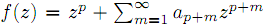which are analytic in the unit disk. By virtue of the Ruscheweyh derivatives we introduce the new subclasses Cp(n,α,β,λ,μ) of Ap. Subordination relations, inclusion relations, convolution properties and a sharp coefficient estimate are obtained. We also give a sufficient condition for a function to be in Cp(n,α,β,λ,μ)

4: Paper Source PDF document

Paper's Title:

Analysis of a Frictional Contact Problem for Viscoelastic Piezoelectric Materials

Author(s):

Meziane Said Ameur, Tedjani Hadj Ammar and Laid Maiza

Departement of Mathematics,
El Oued University,
P.O. Box 789, 39000 El Oued,
Algeria.
E-mail: said-ameur-meziane@univ-eloued.dz

Departement of Mathematics,
El Oued University,
P.O. Box 789, 39000 El Oued,
Algeria.

Department of Mathematics,
Kasdi Merbah University,
30000 Ouargla,
Algeria.
E-mail: maiza.laid@univ-ouargla.dz

Abstract:

In this paper, we consider a mathematical model that describes the quasi-static process of contact between two thermo-electro-viscoelastic bodies with damage and adhesion. The damage of the materials caused by elastic deformations. The contact is frictional and modeled with a normal compliance condition involving adhesion effect of contact surfaces. Evolution of the bonding field is described by a first order differential equation. We derive variational formulation for the model and prove an existence and uniqueness result of the weak solution. The proof is based on arguments of evolutionary variational inequalities, parabolic inequalities, differential equations, and fixed point theorem.

3: Paper Source PDF document

Paper's Title:

A New Hardy-Hilbert's Type Inequality for Double Series and its Applications

Author(s):

Mingzhe Gao

Department of Mathematics and Computer Science, Normal College Jishou University,
Jishou Hunan, 416000,
People's Republic of China
mingzhegao1940@yahoo.com.cn

Abstract:

In this paper, it is shown that a new Hardy-Hilbert’s type inequality for double series can be established by introducing a parameter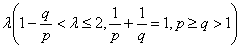and the weight function of the form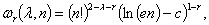where c is Euler constant and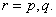And the coefficient is proved to be the best possible. And as the mathematics aesthetics, several important constants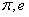and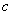appear simultaneously in the coefficient and the weight function when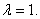In particular, for case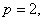some new Hilbert’s type inequalities are obtained. As applications, some extensions of Hardy-Littlewood’s inequality are given.

3: Paper Source PDF document

Paper's Title:

Analysis of the Flow Field in Stenosed Bifurcated Arteries Through a Mathematical Model

Author(s):

S. Chakravarty and S. Sen

Department of Mathematics, Visva-Bharati University,
Santiniketan 731235,
India
santabrata2004@yahoo.co.in

Abstract:

The present study is dealt with an appropriate mathematical model of the arotic bifurcation in the presence of constrictions using which the physiological flow field is analized. The geometry of the bifurcated arterial segment having constrictions in both the parent and its daughter arterial lumen frequently occurring in the diseased arteries causing malfunction of the cardiovascular system , is formed mathematically with the introduction of appropriate curvatures at the lateral junctions and the flow divider. The flowing blood contained in the stenosed bifurcated artery is treated to be Newtonian and the flow is considered to be two dimensional. The motion of the arterial wall and its effect on local fluid mechanics is not ruled out from the present pursuit. The flow analysis applies the time-dependent, two-dimensional incompressible nonlinear Navier-Stokes equations for Newtonian fluid. The flow field can be obtained primarily following the radial coordinate transformation and using the appropriate boundary conditions and finally adopting a suitable finite difference scheme numerically. The influences of the arterial wall distensibility and the presence of stenosis on the flow field, the flow rate and the wall shear stresses are quantified in order to indicate the susceptibility to atherosclerotic lesions and thereby to validate the applicability of the present theoretical model.

3: Paper Source PDF document

Paper's Title:

On Vector Variational Inequality Problem in Terms of Bifunctions

Author(s):

C. S. Lalitha and Monika Mehta

Department of Mathematics, Rajdhani College,
University of Delhi, Raja Garden,
Delhi 110015, India
cslalitha@rediffmail.com

Department of Mathematics, Satyawati College,
University Of Delhi, Ashok Vihar,
Phase-III, Delhi 110052, India
mridul_in@yahoo.com

Abstract:

In this paper, we consider a generalized vector variational inequality problem expressed in terms of a bifunction and establish existence theorems for this problem by using the concepts of cone convexity and cone strong quasiconvexity and employing the celebrated Fan's Lemma. We also give two types of gap functions for this problem.

3: Paper Source PDF document

Paper's Title:

A Subclass of Meromorphically Multivalent Functions with Applications to Generalized Hypergeometric Functions

Author(s):

M. K. Aouf

Mathematics Department, Faculty of Science,
Mansoura University 35516,
Egypt
mkaouf127@yahoo.com

Abstract:

In this paper a new subclass of meromorphically multivalent functions, which is defined by means of a Hadamard product (or convolution) involving some suitably normalized meromorphically p-valent functions. The main object of the present paper is to investigate the various important properties and characteristics of this subclass of meromorphically multivalent functions. We also derive many interesting results for the Hadamard products of functions belonging to this subclass. Also we consider several applications of our main results to generalized hypergeomtric functions.

3: Paper Source PDF document

Paper's Title:

A kind of Function Series and Its Applications

Author(s):

Yang Tianze

Mechanical Engineering,
Shandong University, Xinglongshan Campus,
Jinan, Shandong,
China.

E-mail: qdyangtianze@163.com

Abstract:

A kind of new function series is obtained in this paper. Their theorems and proofs are shown, and some applications are given. We give the expansion form of general integral and the series expansion form of function and the general expansion form of derivative. Using them in the mathematics,we get some unexpected result.

3: Paper Source PDF document

Paper's Title:

Several Applications of a Local Non-convex Young-type Inequality

Author(s):

Loredana Ciurdariu, Sorin Lugojan

Department of Mathematics,
"Politehnica" University of Timisoara,
P-ta. Victoriei, No.2, 300006-Timisoara,
Romania.

E-mail: ltirtirau87@yahoo.com

Abstract:

A local version of the Young inequality for positive numbers is used in order to deduce some inequalities about determinants and norms for real quadratic matrices and norms of positive operators on complex Hilbert spaces.

3: Paper Source PDF document

Paper's Title:

Generalized Von Neumann-Jordan Constant for Morrey Spaces and Small Morrey Spaces

Author(s):

H. Rahman and H. Gunawan

Department of Mathematics,
Islamic State University Maulana Malik Ibrahim Malang,
Jalan Gajayana No.50,
Indonesia.
E-mail: hairur@mat.uin-malang.ac.id

Analysis and Geometry Group,
Faculty of Mathematics and Natural Sciences,
Bandung Institute of Technology, Bandung 40132,
Indonesia.
E-mail: hgunawan@math.itb.ac.id
URL:  http://personal.fmipa.itb.ac.id/hgunawan/

Abstract:

In this paper we calculate some geometric constants for Morrey spaces and small Morrey spaces, namely generalized Von Neumann-Jordan constant, modified Von Neumann-Jordan constants, and Zbaganu constant. All these constants measure the uniformly nonsquareness of the spaces. We obtain that their values are the same as the value of Von Neumann-Jordan constant for Morrey spaces and small Morrey spaces.

2: Paper Source PDF document

Paper's Title:

Monotonicity Properties for Generalized Logarithmic Means

Author(s):

Chao-Ping Chen and Feng Qi

Department of Applied Mathematics and Informatics, Research Institute of Applied Mathematics,
Henan Polytechnic University, Jiaozuo City, Henan 454000, China

chenchaoping@hpu.edu.cn

Department of Applied Mathematics and Informatics, Research Institute of Applied Mathematics,
Henan Polytechnic University, Jiaozuo City, Henan 454000, China

qifeng@hpu.edu.cn, fengqi618@member.ams.org

U
rl: http://rgmia.vu.edu.au/qi.html, http://dami.hpu.edu.cn/qifeng.html

Abstract:

In this paper, we consider the monotonicity properties for ratio of two generalized logarithmic means, and then use it to extend and complement a recently published result of F. Qi and B.-N. Guo.

2: Paper Source PDF document

Paper's Title:

Iterated Order of Fast Growth Solutions of Linear Differential Equations

Author(s):

Benharrat Belaďdi

Department of Mathematics
Laboratory of Pure and Applied Mathematics
University of Mostaganem
B. P. 227 Mostaganem,
ALGERIA.
belaidi@univ-mosta.dz

Abstract:

In this paper, we investigate the growth of solutions of the differential equation f(k) + Ak-1 (z) f(k-1) +...+ A1 (z) f' + A0 (z) f= F (z), where Ao (z), ..., Ak-1 (z) and F (z)0 are entire functions. Some estimates are given for the iterated order of solutions of the above quation when one of the coefficients As is being dominant in the sense that it has larger growth than Aj (j≠s) and F.

2: Paper Source PDF document

Paper's Title:

Positive Solutions for Systems of Three-point Nonlinear Boundary Value Problems

Author(s):

J. Henderson and S. K. Ntouyas

Department of Mathematics, Baylor University
Waco, Texas
76798-7328 USA.
Johnny_Henderson@baylor.edu
URL: http://www3.baylor.edu/~Johnny_Henderson

Department of Mathematics, University of Ioannina
451 10 Ioannina,
Greece.
sntouyas@cc.uoi.gr
URL: http://www.math.uoi.gr/~sntouyas

Abstract:

Values of λ are determined for which there exist positive solutions of the system of three-point boundary value problems, u''(t)+ λa(t)f(v(t))=0, v''(t)+λb(t)g(u(t))=0, for 0 < t <1, and satisfying, u(0) = 0, u(1)=α u(η), v(0) = 0, v(1)=α v(η). A Guo-Krasnosel'skii fixed point theorem is applied.

2: Paper Source PDF document

Paper's Title:

Fixed Points and Stability of the Cauchy Functional Equation

Author(s):

Choonkil Park and Themistocles M. Rassias

Department of Mathematics, Hanyang University,
Seoul 133-791,
Republic of Korea

Department of Mathematics, National Technical University of Athens,
Zografou Campus, 15780 Athens,
Greece

baak@hanyang.ac.kr
trassias@math.ntua.gr

Abstract:

Using fixed point methods, we prove the generalized Hyers-Ulam stability of homomorphisms in Banach algebras and of derivations on Banach algebras for the Cauchy functional equation.

2: Paper Source PDF document

Paper's Title:

Generalized Efficient Solutions to One Class of Vector Optimization Problems in Banach Space

Author(s):

Peter I. Kogut, Rosanna Manzo, and Igor V. Nechay

Department of Differential Equations,
Dnipropetrovsk National University,
Naukova str., 13, 49050 Dnipropetrovsk,
Ukraine
p.kogut@i.ua

Dipartimento di Ingegneria Dell’informazione e Matematica Applicata,
Universitŕ di Salerno,
Via Ponte Don Melillo, 84084 Fisciano (Sa),
Italy
manzo@diima.unisa.it

Department of Technical Cybernetics,
Dnipropetrovsk Technical University,
Dnipro
petrovsk,
Ukraine
i.nechay@i.ua

Abstract:

In this paper, we study vector optimization problems in Banach spaces for essentially nonlinear operator equations with additional control and state constraints. We assume that an objective mapping possesses a weakened property of lower semicontinuity and make no assumptions on the interior of the ordering cone. Using the penalization approach we derive both sufficient and necessary conditions for the existence of efficient solutions of the above problems. We also prove the existence of the so-called generalized efficient solutions via the scalarization of some penalized vector optimization problem.

2: Paper Source PDF document

Paper's Title:

Bounds for Two Mappings Associated to the Hermite-Hadamard Inequality

Author(s):

S. S. Dragomir1,2 and I. Gomm1

1Mathematics, School of Engineering & Science,
Victoria University,
PO Box 14428, Melbourne City, MC 8001,
Australia.

2School of Computational & Applied Mathematics,
University of the Witwatersrand,
Private Bag 3, Johannesburg 2050,
South Africa.

sever.dragomir@vu.edu.au
ian.gomm@vu.edu.au
URL: http://rgmia.org/dragomir

Abstract:

Some inequalities concerning two mappings associated to the celebrated Hermite-Hadamard integral inequality for convex function with applications for special means are given.

2: Paper Source PDF document

Paper's Title:

Approximation of an AQCQ-Functional Equation and its Applications

Author(s):

Choonkil Park and Jung Rye Lee

Department of Mathematics,
Research Institute for Natural Sciences,
Hanyang University, Seoul 133-791,
Korea;

Department of Mathematics,
Daejin University,
Kyeonggi 487-711,
Korea

baak@hanyang.ac.kr
jrlee@daejin.ac.kr

Abstract:

This paper is a survey on the generalized Hyers-Ulam stability of an AQCQ-functional equation in several spaces. Its content is divided into the following sections:

1. Introduction and preliminaries.

2. Generalized Hyers-Ulam stability of an AQCQ-functional equation in Banach spaces: direct method.

3. Generalized Hyers-Ulam stability of an AQCQ-functional equation in Banach spaces: fixed point method.

4. Generalized Hyers-Ulam stability of an AQCQ-functional equation in random Banach spaces: direct method.

5. Generalized Hyers-Ulam stability of an AQCQ-functional equation in random Banach spaces: fixed point method.

6. Generalized Hyers-Ulam stability of an AQCQ-functional equation in non-Archi-medean Banach spaces: direct method.

7. Generalized Hyers-Ulam stability of an AQCQ-functional equation in non-Archi-medean Banach spaces: fixed point method.

2: Paper Source PDF document

Paper's Title:

Finite and Infinite Order Solutions of a Class of Higher Order Linear Differential Equations

Author(s):

Department of Mathematics,
Laboratory of Pure and Applied Mathematics,
University of Mostaganem, B. P 227 Mostaganem,
ALGERIA

Abstract:

In this paper, we investigate the growth of solutions of higher order linear differential equations where most of the coefficients have the same order and type with each other.

2: Paper Source PDF document

Paper's Title:

Further Bounds for Two Mappings Related to the Hermite-Hadamard Inequality

Author(s):

S. S. Dragomir1,2 and I. Gomm1

1Mathematics, School of Engineering & Science,
Victoria University,
PO Box 14428, Melbourne City, MC 8001,
Australia.

2School of Computational & Applied Mathematics,
University of the Witwatersrand,
Private Bag 3, Johannesburg 2050,
South Africa.

sever.dragomir@vu.edu.au
ian.gomm@vu.edu.au
URL: http://rgmia.org/dragomir

Abstract:

Some new results concerning two mappings associated to the celebrated Hermite-Hadamard integral inequality for twice differentiable functions with applications for special means are given.

2: Paper Source PDF document

Paper's Title:

Positive Solutions to a System of Boundary Value Problems for Higher-Dimensional Dynamic Equations on Time Scales

Author(s):

I. Y. Karaca

Department of Mathematics,
Ege University,
35100 Bornova, Izmir,
Turkey

ilkay.karaca@ege.edu.tr

URL: http://ege.edu.tr

Abstract:

In this paper, we consider the system of boundary value problems for higher-dimensional dynamic equations on time scales. We establish criteria for the existence of at least one or two positive solutions. We shall also obtain criteria which lead to nonexistence of positive solutions. Examples applying our results are also given.

2: Paper Source PDF document

Paper's Title:

Some Applications of Fejér's Inequality for Convex Functions (I)

Author(s):

S.S. Dragomir1,2 and I. Gomm1

1Mathematics, School of Engineering & Science
Victoria University, PO Box 14428
Melbourne City, MC 8001,
Australia.

sever.dragomir@vu.edu.au

URL: http://rgmia.org/dragomir

2School of Computational & Applied Mathematics,
University of the Witwatersrand,
Private Bag 3, Johannesburg 2050,
South Africa.

Abstract:

Some applications of Fejér's inequality for convex functions are explored. Upper and lower bounds for the weighted integral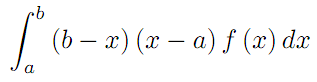under various assumptions for f with applications to the trapezoidal quadrature rule are given. Some inequalities for special means are also provided

2: Paper Source PDF document

Paper's Title:

Inequalities for the Area Balance of Functions of Bounded Variation

Author(s):

Sever S. Dragomir1,2

1Mathematics, School of Engineering & Science
Victoria University, PO Box 14428
Melbourne City, MC 8001,
Australia
E-mail: sever.dragomir@vu.edu.au

2DST-NRF Centre of Excellence in the Mathematical and Statistical Sciences,
School of Computer Science & Applied Mathematics,
University of the Witwatersrand,
Private Bag 3, Johannesburg 2050,
South Africa
URL: http://rgmia.org/dragomir

Abstract:

We introduce the area balance function associated to a Lebesgue integrable function f:[a,b] C by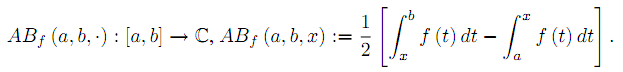Several sharp bounds for functions of bounded variation are provided. Applications for Lipschitzian and convex functions are also given.

2: Paper Source PDF document

Paper's Title:

MSplit Equality for Monotone Inclusion Problem and Fixed Point Problem in Real Banach Spaces

Author(s):

1,2Christian Chibueze Okeke, 3Abdumalik Usman Bello, 1Chinedu Izuchukwu, and 1Oluwatosin Temitope Mewomo

1School of Mathematics,
Statistics and Computer Science,
University of KwaZulu-Natal, Durban,
South Africa.
E-mail: okekec@ukzn.ac.za
E-mail: izuchukwuc@ukzn.ac.za
E-mail: mewomoo@ukzn.ac.za

2DST-NRF Center of Excellence in Mathematical and Statistical Sciences (CoE-Mass)
Johannesburg,
South Africa.

3Federal University,
Dutsin-Ma, Katsina State,
Nigeria.
E-mail: uabdulmalik@fudutsinma.edu.ng

Abstract:

In this paper a new iterative algorithm for approximating a common solution of split equality monotone inclusion problem and split equality fixed point problem is introduced. Using our algorithm, we state and prove a strong convergence theorem for approximating an element in the intersection of the set of solutions of a split equality monotone inclusion problem and the set of solutions of a split equality fixed point problem for right Bregman strongly nonexpansive mappings in the setting of p-uniformly convex Banach spaces which are also uniformly smooth. We also give some applications.

2: Paper Source PDF document

Paper's Title:

A Low Order Least-Squares Nonconforming Finite Element Method for Steady Magnetohydrodynamic Equations

Author(s):

Z. Yu, D. Shi and H. Zhu

College of Science,
Zhongyuan University of Technology,
Zhengzhou 450007,
China.
E-mail: 5772@zut.edu.cn

School of Mathematics and Statistics,
Zhengzhou University,
Zhengzhou 450001,
China.
E-mail: shi_dy@126.com

Mathematics Department,
University of Southern Mississippi,
Hattiesburg MS, 39406,
U.S.A
E-mail: huiqing.zhu@usm.edu

Abstract:

A low order least-squares nonconforming finite element (NFE) method is proposed for magnetohydrodynamic equations with EQ1rot element and zero-order Raviart-Thomas element. Based on the above element's typical interpolations properties, the existence and uniqueness of the approximate solutions are proved and the optimal order error estimates for the corresponding variables are derived.

1: Paper Source PDF document

Paper's Title:

Fekete-Szegö Inequality for Certain Class of Analytic Functions

Author(s):

V. Ravichandran, Maslina Darus, M. Hussain Khan, and  K. G. Subramanian

School of Mathematical Sciences, Universiti Sains Malaysia,
11800 Usm, Penang, Malaysia

School of Mathematical Sciences, Faculty of Sciences and Technology,
Ukm, Banki 43600, Malaysia

Department of Mathematics, Islamiah College,
V

Department of Mathematics, Madras Christian College, Tambaram,
Chennai- 600 059, India

Abstract:

In this present investigation, the authors obtain Fekete-Szegö inequality for a certain class of analytic functions f(z) for which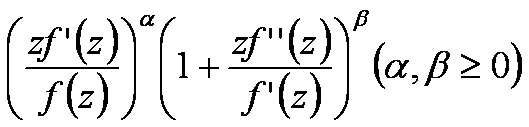lies in a region starlike with respect to 1 and symmetric with respect to the real axis. Also certain application of our main result for a class of functions defined by Hadamard product (convolution) is given. As a special case of our result we obtain Fekete-Szegö inequality for a class of functions defined through fractional derivatives. Also we obtain Fekete-Szegö inequality for the inverse functions.

1: Paper Source PDF document

Paper's Title:

A Simple New Proof of Fan-Taussky-Todd Inequalities

Author(s):

Zhi-Hua Zhang and Zhen-Gang Xiao

Zixing Educational Research Section,
Chenzhou City, Hunan 423400, P. R. China.
Zhi-hua Zhang
Url: http://www.hnzxslzx.com/zzhweb/

Department Of Mathematics, Hunan Institute Of Science And Technology,
Yueyang City, Hunan 423400, P. R. China.
Zhen-gang Xiao

Abstract:

In this paper we present simple new proofs of the inequalities: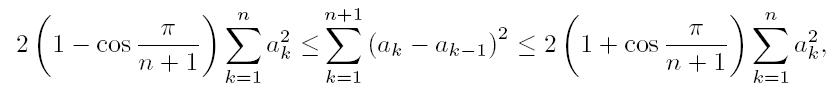which holds for all real numbers a0 = 0, a1, · · · , an, an+1 = 0 and the coefficients 2(1 - cos(π/(n + 1))) and 2(1 + cos(π/(n + 1))) are the best possible; and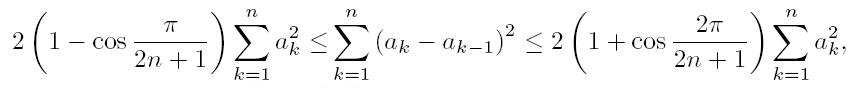which holds for all real numbers a0 = 0, a1, · · · , an and the coefficients 2(1-cos(π/(2n + 1))) and 2(1 + cos(π/(2n + 1))) are the best possible.

1: Paper Source PDF document

Paper's Title:

Two Mappings Related to Steffensen's Inequalities

Author(s):

Liang-Cheng Wang

School of Mathematical Science,
Chongqing Institute of Technology,
Xingsheng Lu 4,
Yangjiaping 400050, Chongqing City,
China.
wangliangcheng@163.com

Abstract:

In this paper, we define two mappings closely connected with Steffensen's inequalities, investigate their main properties, give some refinements for Steffensen's inequalities and obtain new inequalities.

1: Paper Source PDF document

Paper's Title:

On the Fekete-Szegő Inequality for Some Subclasses of Analytic Functions

Author(s):

T.N. Shanmugam and A. Singaravelu

Department of Mathematics,
College of Engineering,
Anna University, Chennai-600 025,
shan@annauniv.edu

Department of Mathematics,
Valliammai Engineering College,
Chennai-603 203,
sivasaisastha@rediffmail.com

Abstract:

In this present investigation, the authors obtainFekete-Szegő's inequality for certain normalized analytic functions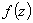defined on the open unit disk for which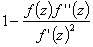lie in a region starlike with respect to 1 and symmetric with respect to the real axis. Also certain applications of the main result for a class of functions defined by convolution are given. As a special case of this result, Fekete-Szegő's inequality for a class of functions defined through fractional derivatives is also obtained.

1: Paper Source PDF document

Paper's Title:

A Nonlinear Proximal Alternating Directions Method for Structured Variational Inequalities

Author(s):

M. Li

Department of Management Science and Engineering, School of Economics and Management
Southeast University, Nanjing, 210096,
China.
liminnju@yahoo.com

Abstract:

In this paper, we present a nonlinear proximal alternating directions method (NPADM) for solving a class of structured variational inequalities (SVI). By choosing suitable Bregman functions, we generalize the proximal alternating directions method proposed by He, et al.. The convergence of the method is proved under quite mild assumptions and flexible parameter conditions.

1: Paper Source PDF document

Paper's Title:

Fekete-Szegö Problem for Univalent Functions with Respect to k-Symmetric Points

Author(s):

K. Al-Shaqsi and M. Darus

School of Mathematical Sciences, Faculty of Science and Technology,
University Kebangsaan Malaysia,
Bangi 43600 Selangor D. Ehsan,
Malaysia
ommath@hotmail.com
maslina@ukm.my

Abstract:

In the present investigation, sharp upper bounds of |a3- μa22| for functions f(z) = z + a2z2 + a2z3 + ... belonging to certain subclasses of starlike and convex functions with respect to k-symmetric points are obtained. Also certain applications of the main results for subclasses of functions defined by convolution with a normalized analytic function are given. In particular, Fekete- Szegö inequalities for certain classes of functions defined through fractional derivatives are obtained.

1: Paper Source PDF document

Paper's Title:

Uniqueness of Meromorphic Functions and Weighted Sharing

Author(s):

Indrajit Lahiri and Rupa Pal

Department of Mathematics, University of
Kalyani, West Bengal 741235, India

Jhargram Raj College,
Jhargram, Midnapur(W),
West Bengal 721507,
India
rupa.a.pal@gmail.com

Abstract:

With the help of the notion of weighted sharing of values, we prove a result on uniqueness of meromorphic functions and as a consequence we improve a result of P. Li

1: Paper Source PDF document

Paper's Title:

Topological Aspects of Scalarization in Vector Optimization Problems.

Author(s):

Peter I. Kogut, Rosanna Manzo and Igor V. Nechay

Department of Differential Equations,
Dnipropetrovsk National University, Naukova STR.,
13, 49010 Dnipropetrovsk,
Ukraine

p.kogut@i.ua

Universitŕ di Salerno,
Dipartimento di Ingegneria dell'Informazione e Matematica Applicata,
Via Ponte don Melillo, 84084 Fisciano (SA),
Italy

manzo@diima.unisa.it

Department of Technical Cybernetics,
Dnipropetrovsk Technical University,
Acad. Lazarjan STR., 2, 49010 Dnipropetrovsk,
Ukraine

i.nechay@i.ua

Abstract:

In this paper, we study vector optimization problems in partially ordered Banach spaces. We suppose that the objective mapping possesses a weakened property of lower semicontinuity and make no assumptions on the interior of the ordering cone. We derive sufficient conditions for existence of efficient solutions of the above problems and discuss the role of topological properties of the objective space. We discuss the scalarization of vector optimization problems when the objective functions are vector-valued mappings with a weakened property of lower semicontinuity. We also prove the existence of the so-called generalized efficient solutions via the scalarization process. All principal notions and assertions are illustrated by numerous examples.

1: Paper Source PDF document

Paper's Title:

The Superstability of the Pexider Type Trigonometric Functional Equation

Author(s):

Gwang Hui Kim and Young Whan Lee

Department of Mathematics, Kangnam
University Yongin, Gyeonggi, 446-702, Korea.
ghkim@kangnam.ac.kr

Department of Computer and Information Security
Daejeon University, Daejeon 300-716, Korea.
ywlee@dju.ac.kr

Abstract:

The aim of this paper is to investigate the stability problem for
the Pexider type (hyperbolic) trigonometric functional equation
f(x+y)+f(x+σy)=λg(x)h(y) under the conditions :
|f(x+y)+f(x+σy)- λg(x)h(y)|≤φ(x),
φ(y)
, and min {φ(x), φ (y)}.

As a consequence, we have generalized the results of stability for
the cosine(d'Alembert), sine, and the Wilson functional equations by J.
Baker, P. Găvruta, R. Badora and R. Ger, Pl.~Kannappan, and G.
H. Kim

1: Paper Source PDF document

Paper's Title:

Inclusion Properties of a Certain Subclass of Strongly Close-To-Convex Functions

Author(s):

S. M. Khairnar and M. More

Department of Mathematics,
Alandi -412 105, Pune, Maharashtra,
INDIA.

smkhairnar2007@gmail.com, meenamores@gmail.com.

Abstract:

The purpose of this paper is to derive some inclusion and argument properties of a new subclass of strongly close-to-convex functions in the open unit disc. We have considered an integral operator defined by convolution involving hypergeometric function in the subclass definition. The subclass also extends to the class of α-spirallike functions of complex order.

1: Paper Source PDF document

Paper's Title:

Some Distortion and Other Properties Associated with a Family of the n-Fold Symmetric Koebe Type Functions

Author(s):

H. M. Srivastava, N. Tuneski and E. Georgieva-Celakoska

Department of Mathematics and Statistics, University of Victoria,
Victoria, British Columbia V8W 3R4,

harimsri@math.uvic.ca

Faculty of Mechanical Engineering, St.
Cyril and Methodius University,
Karpo'v s II b.b., MK-1000 Skopje,
Republic of Macedonia

Abstract:

In a recent work by Kamali and Srivastava , a certain family of the n-fold symmetric Koebe type functions was introduced and studied systematically. In an earlier investigation, Eguchi and Owa  had considered its special case when n=1 (see also ). Here, in our present sequel to these earlier works, this general family of the n-fold symmetric Koebe type functions is studied further and several distortion theorems and such other properties as the radii of spirallikeness, the radii of starlikeness and the radii of convexity, which are associated with this family of the n-fold symmetric Koebe type functions, are obtained. We also provide certain criteria that embed this family of the n-fold symmetric Koebe type functions in a function class Gλ which was introduced and studied earlier by Silverman .

1: Paper Source PDF document

Paper's Title:

On Some Estimates for the Logarithmic Mean

Author(s):

Department of Information and Computer Engineering,
Kisarazu National College of Technology,
Kisarazu, Chiba 292-0041,
Japan.

Abstract:

We show some estimates for the logarithmic mean that are obtained from operator inequalities between the Barbour path and the Heinz means.

1: Paper Source PDF document

Paper's Title:

Hermite-Hadamard-Fejer Type Inequalities for Harmonically s-convex Functions via Fractional Integrals

Author(s):

İmdat İşcan, Mehmet Kunt

Department of Mathematics,
Faculty of Sciences and Arts,
Giresun University, Giresun,
Turkey.
E-mail: imdat.iscan@giresun.edu.tr

Department of Mathematics,
Faculty of Sciences,
61080, Trabzon,
Turkey.
E-mail: mkunt@ktu.edu.tr

Abstract:

In this paper, some Hermite-Hadamard-Fejer type integral inequalities for harmonically s-convex functions in fractional integral forms have been obtained.

1: Paper Source PDF document

Paper's Title:

Some New Generalizations of Jensen's Inequality with Related Results and Applications

Author(s):

Steven G. From

Department of Mathematics

E-mail: sfrom@unomaha.edu

Abstract:

In this paper, some new generalizations of Jensen's inequality are presented. In particular, upper and lower bounds for the Jensen gap are given and compared analytically and numerically to previously published bounds for both the discrete and continuous Jensen's inequality cases. The new bounds compare favorably to previously proposed bounds. A new method based on a series of locally linear interpolations is given and is the basis for most of the bounds given in this paper. The wide applicability of this method will be demonstrated. As by-products of this method, we shall obtain some new Hermite-Hadamard inequalities for functions which are 3-convex or 3-concave. The new method works to obtain bounds for the Jensen gap for non-convex functions as well, provided one or two derivatives of the nonlinear function are continuous. The mean residual life function of applied probability and reliability theory plays a prominent role in construction of bounds for the Jensen gap. We also present an exact integral representation for the Jensen gap in the continuous case. We briefly discuss some inequalities for other types of convexity, such as convexity in the geometric mean, and briefly discuss applications to reliability theory.

1: Paper Source PDF document

Paper's Title:

Ostrowski Type Fractional Integral Inequalities for Generalized (s,m,φ)-preinvex Functions

Author(s):

Artion Kashuri and Rozana Liko

University of Vlora "Ismail Qemali",
Faculty of Technical Science,
Department of Mathematics, 9400,
Albania.
E-mail: artionkashuri@gmail.com
E-mail: rozanaliko86@gmail.com

Abstract:

In the present paper, the notion of generalized (s,m,φ)-preinvex function is introduced and some new integral inequalities for the left hand side of Gauss-Jacobi type quadrature formula involving generalized (s,m,φ)-preinvex functions along with beta function are given. Moreover, some generalizations of Ostrowski type inequalities for generalized (s,m,φ)-preinvex functions via Riemann-Liouville fractional integrals are established.

1: Paper Source PDF document

Paper's Title:

Dynamical Analysis of HIV/AIDS Epidemic Model with Two Latent Stages, Vertical Transmission and Treatment

Author(s):

Nur Shofianah, Isnani Darti, Syaiful Anam

Mathematics Department,Faculty of Mathematics and Natural Sciences.
University of Brawijaya,
Jl. Veteran, Malang 65145,
Indonesia.
E-mail: nur_shofianah@ub.ac.id, isnanidarti@ub.ac.id, syaiful@ub.ac.id

Abstract:

We discuss about dynamical analysis of HIV/AIDS epidemic model with two latent stages, vertical transmission and treatment. In this model, the spreading of HIV occurs through both horizontal and vertical transmission. There is also treatment for individual who has been HIV infected. The latent stage is divided into slow and fast latent stage based on the immune condition which varies for each individual. Dynamical analysis result shows that the model has two equilibrium points: the disease-free equilibrium point and the endemic equilibrium point. The existence and global stability of equilibrium points depend on the basic reproduction number R0. When R0 <1, only the disease-free equilibrium point exists. If R0 >1, there are two equilibrium points, which are the disease-free equilibrium point and the endemic equilibrium point. Based on the result of stability analysis, the disease-free equilibrium point is globally asymptotically stable if R0 <1, while if R0 > 1 and p=q, the endemic equilibrium point will be globally asymptotically stable. In the end, we show some numerical simulations to support the analytical result.

1: Paper Source PDF document

Paper's Title:

Composite Variational-Like Inequalities Given By Weakly Relaxed ζ-Semi-Pseudomonotone Multi-Valued Mapping

Author(s):

Syed Shakaib Irfan, Iqbal Ahmad, Zubair Khan and Preeti Shukla

College of Engineering, Qassim University
Buraidah, Al-Qassim,
Saudi Arabia.
E-mail: shakaib@qec.edu.sa

College of Engineering, Qassim University
Buraidah, Al-Qassim,
Saudi Arabia.
E-mail: iqbal@qec.edu.sa

Department of Mathematics,
Integral University Lucknow,
India.
E-mail: zkhan@iul.ac.in

Department of Mathematics,
Integral University Lucknow,
India.
E-mail: shuklapreeti1991@gmail.com

Abstract:

In this article, we introduce a composite variational-like inequalities with weakly relaxed ζ-pseudomonotone multi-valued maping in reflexive Banach spaces. We obtain existence of solutions to the composite variational-like inequalities with weakly relaxed ζ-pseudomon -otone multi-valued maps in reflexive Banach spaces by using KKM theorem. We have also checked the solvability of the composite variational-like inequalities with weakly relaxed ζ-semi-pseudomonotone multi-valued maps in arbitrary Banach spaces using Kakutani-Fan-Glicksberg fixed point theorem.

1: Paper Source PDF document

Paper's Title:

Double Difference of Composition Operator on Bloch Spaces

Author(s):

Rinchen Tundup

Department of Mathematics
University of Jammu
Jammu and Kashmir
India.

E-mail: joneytun123@gmail.com

Abstract:

In this paper we characterize the compactness of double difference of three non-compact composition operators on Bloch space induced by three holomorphic self maps on the unit disc.

1: Paper Source PDF document

Paper's Title:

Author(s):

F. U. Ogbuisi

School of Mathematics, Statistics and Computer Science,
University of KwaZulu-Natal, Durban,
South Africa.

Department of Mathematics,
University of Nigeria, Nsukka,
Nigeria.
E-mail: ferdinard.ogbuisi@unn.edu.ng fudochukwu@yahoo.com

Abstract:

In this paper, we propose and analyze a type of subgradient extragradient algorithm for the approximation of a solution of variational inequality problem which is also a common fixed point of an infinite family of relatively nonexpansive mappings in 2-uniformly convex Banach spaces which are uniformly smooth. By using the generalized projection operator, we prove a strong convergence theorem which does not require the prior knowledge of the Lipschitz constant of cost operator. We further applied our result to constrained convex minimization problem, convex feasibility problem and infinite family of equilibrium problems. Our results improve and complement related results in 2-uniformly convex and uniformly smooth Banach spaces and Hilbert spaces.

1: Paper Source PDF document

Paper's Title:

Reduced Generalized Combination Synchronization Between Two n-Dimensional Integer-Order Hyperchaotic Systems and One m-Dimensional Fractional-Order Chaotic System

Author(s):

Smail Kaouache, Mohammed Salah Abdelouahab and Rabah Bououden

Laboratory of Mathematics and their interactions,
Abdelhafid Boussouf University Center, Mila.
Algeria
E-mail: smailkaouache@gmail.com, medsalah3@yahoo.fr, rabouden@yahoo.fr

Abstract:

This paper is devoted to investigate the problem of reduced generalized combination synchronization (RGCS) between two n-dimensional integer-order hyperchaotic drive systems and one m-dimensional fractional-order chaotic response system. According to the stability theorem of fractional-order linear system, an active mode controller is proposed to accomplish this end. Moreover, the proposed synchronization scheme is applied to synchronize three different chaotic systems, which are the Danca hyperchaotic system, the modified hyperchaotic Rossler system, and the fractional-order Rabinovich-Fabrikant chaotic system. Finally, numerical results are presented to fit our theoretical analysis.

1: Paper Source PDF document

Paper's Title:

Analysis of the Dynamic Response of the Soil-pile Behavioral Model Under Lateral Load

Author(s):

Ibrahima Mbaye, Mamadou Diop, Aliou Sonko and Malick Ba

University of Thies,
Department of Mathematics, Bp 967 Thies,
Senegal.
E-mail: imbaye@univ-thies.sn
aliousonko59@gmail.com
mmalickba@hotmail.fr
URL: https://www.univ-thies.sn

Abstract:

This work aims to extend and improve our previous study on mathematical and numerical analysis of stationary Pasternak model. In this paper a dynamic response of Pasternak model is considered. On the one hand we establish the existence and uniqueness of the solution by using the Lax-Milgram theorem and the spectral theory thus the existence of a Hilbert basis is shown and the spectral decomposition of any solution of the problem can be established and on the other hand the finite element method is used to determinate the numerical results. Furthermore, the influence of soil parameters Gp and Kp on the displacement of the pious is studied numerically at any time tn.

1: Paper Source PDF document

Paper's Title:

Coexisting Attractors and Bubbling Route to Chaos in Modified Coupled Duffing Oscillators

Author(s):

B. Deruni1, A. S. Hacinliyan1,2, E. Kandiran3, A. C. Keles2, S. Kaouache4, M.-S. Abdelouahab4, N.-E. Hamri4

1Department of Physics,
University of Yeditepe,
Turkey.

2Department of Information Systems and Technologies,
University of Yeditepe,
Turkey

3Department of Software Development,
University of Yeditepe,
Turkey.

4Laboratory of Mathematics and their interactions,
University Center of Abdelhafid Boussouf,
Mila 43000,
Algeria.

E-mail: berc890@gmail.com
ahacinliyan@yeditepe.edu.tr
engin.kandiran@yeditepe.edu.tr
cihan.keles@yeditepe.edu.tr
s.kaouache@centr-univ-mila.dz
medsalah3@yahoo.fr
n.hamri@centre-univ-mila.dz

Abstract:

In this article dynamical behavior of coupled Duffing oscillators is analyzed under a small modification. The oscillators have cubic damping instead of linear one. Although single duffing oscillator has complex dynamics, coupled duffing systems possess a much more complex structure. The dynamical behavior of the system is investigated both numerically and analytically. Numerical results indicate that the system has double scroll attractor with suitable parameter values. On the other hand, bifurcation diagrams illustrate rich behavior of the system, and it is seen that, system enters into chaos with different routes. Beside classical bifurcations, bubbling route to chaos is observed for suitable parameter settings. On the other hand, Multistability of the system is indicated with the coexisting attractors, such that under same parameter setting the system shows different periodic and chaotic attractors. Moreover, chaotic synchronization of coupled oscillators is illustrated in final section.

Search and serve lasted 1 second(s).